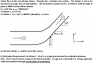# Check Fluid Flow Through Pipe: Calculate Pressure of P1

• pyroknife

#### pyroknife

I already got the answer verified for part a which is v1=1.5 m/s and mass flow rate=23.1 kg/s

For part b, I'd like someone to check my answer and answer a question.

Sum of forces in the x direction ƩFx=m'out * V2 - m'in * V1
m'=mass flow rate
V=velocity

For the left hand side of the equation gives:
ƩFx=-Rx + P1*A1 - P2*A2*cos(50)

Can someone explain why the force due to the pressure @ 2 causes a negative force in the x direction?

=-3880+P1*pi*(.07^2) - 199*pi(.035^2)cos50
I think my professor said that we need to use the gauge pressure in this conservation of momentum equation, thus P2=300-101=199kPa

Fort the right hand side of the equation:
density*V2*A2*cos(50)*V2 - density*V1*A1*V1=1000*6*PI*(.035^2)*COS(50)*6-1000*1.5*pi*(.07^2)*1.5

P1=256 kPa (gage pressure)

#### Attachments

•Untitled.png
10.9 KB · Views: 412
"Can someone explain why the force due to the pressure @ 2 causes a negative force in the x direction?"

If you draw a control volume around the section of pipe, the pressure on the control volume at position 2 where fluid leaves creates a force that points to the left (and down) which is the negative x direction. At position 1 where the fluid enters the control volume, the force due to the pressure is to the right which would be positive.

I tried drawing the control volume, but I'm still not seeing why one would be positive and one would be negative.

Can I think of it this way:
@1 The arrow points into the pipe,thus (+) force.
@2 the arrow points away from the pipe, thus (-) force.

I tried drawing the control volume, but I'm still not seeing why one would be positive and one would be negative.

Can I think of it this way:
@1 The arrow points into the pipe,thus (+) force.
@2 the arrow points away from the pipe, thus (-) force.

One way to keep it straight with respect to the coordinate system chosen (one is already assigned in this problem) is to put all terms associated with input on one side of the equation. They will all be positive if the flow is in the same direction of the coordinate system. Put all the terms describing the exiting flow on the other side of the equals mark keeping the coordinate system in mind. That makes the pressure and velocity terms negative when brought across the equals mark.